# 220V Half Wave Controlled Rectifier with Arduino

This post shows how to build a half wave controlled rectifier using Arduino uno board and one thyristor (SCR).
No warranty is provided with this project, do it at your own risk!
SCR: Silicon Controlled Rectifier.

The SCR (thyristor) is a three-terminal device (Anode, Cathode and Gate) with four layers of alternating p- and n-type material. The gate terminal is used to control the SCR, the anode (A) and cathode (K) are connected in series with the load. The SCR is just a controlled diode.

In single-phase half-wave rectifier, only one thyristor is used to control the load voltage. The thyristor will conduct (ON state) when the voltage Vt is positive (Vt > 0) and a firing current pulse Ig is applied to the gate terminal. Delaying the firing pulse by an angle ‘alpha’ does the control of the load voltage. In the figure below the angle ‘alpha’ is measured from the zero crossing point of the supply voltage Vs. The load is resistive and therefore current id has the same waveform as the load voltage. The thyristor goes to the nonconducting condition (OFF state) when the load voltage and, consequently, the current try to reach a negative value.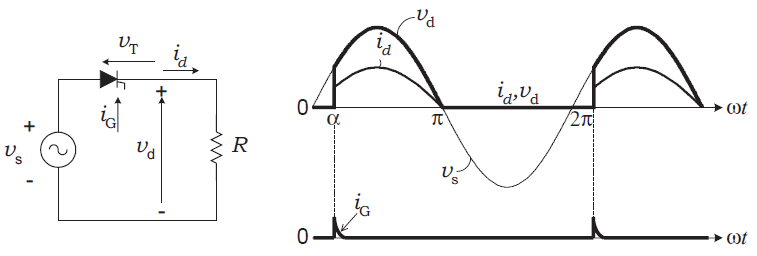220V Half wave controlled rectifier with Arduino circuit:
The following image shows project hardware circuit diagram.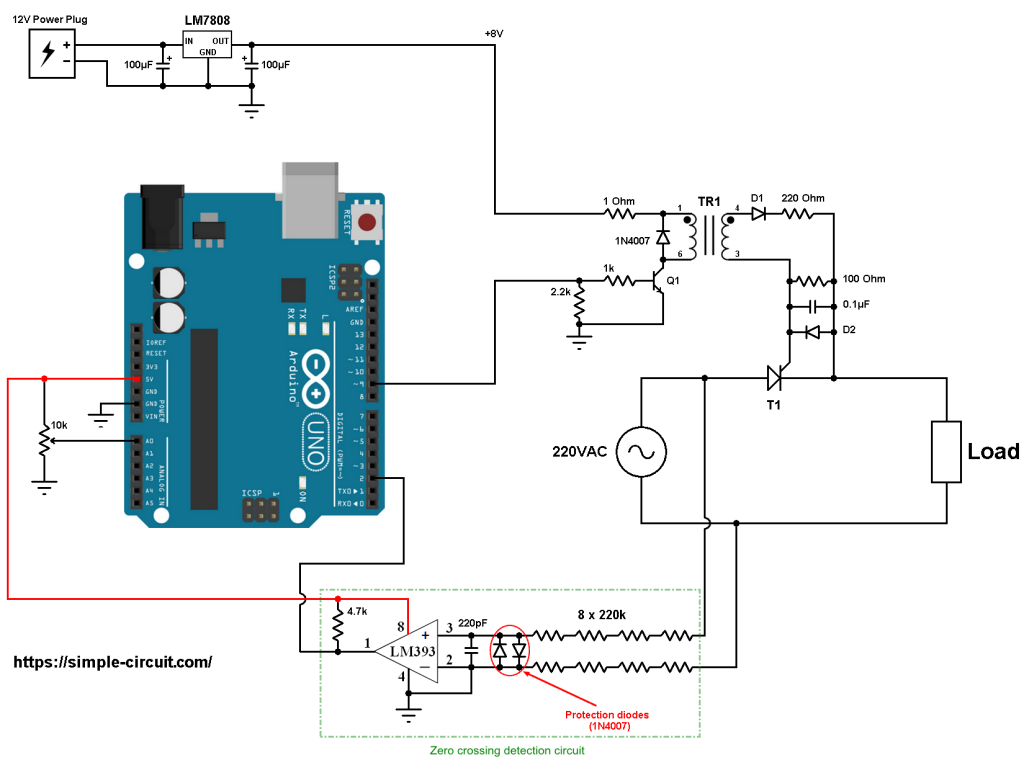All the grounded terminals are connected together.

The input voltage of the circuit is 220V/230V 50Hz alternating current (AC) from home outlet. The phase (line) terminal is connected to thyristor T1 anode and the natural is connected directly to the load.
The load can be purely resistive (for example lamp) or inductive (motor).
I’ve used a simple lamp with 40W (purely resistive load).

The cathode of the thyristor is directly connected to the load.
In this project I used TYN1225 thyristor (datasheet).

The transformer TR1 is pulse train transformer which is used just for firing the SCR. Its full name is: KMB472/101 (KMB472-101) from YHDC (datasheet).
The KMB472-101 works with voltage of 8V, so to get 8V I used LM7808 voltage regulator. The LM7808 gives a regulated 8V from an external power supply of 12V.
The KMB472-101 is connected to the LM7808 regulator (8V) through a resistor of 1 ohm.

The diodes D1 and D2 are simple diodes which may be 1N4007 or 1N4148.
The transistor Q1 is an NPN transistor which may be KSC2383 (datasheet) or equivalent. I’m using the KSC2383 in my hardware circuit!
The Q1 transistor base terminal is connected to Arduino pin 9 through 1k ohm resistor.

In this project I used the LM393 (dual comparator IC) for the zero crossing events detection, an optocoupler can be used for the same purpose but I think the comparator is much better because it gives an exact results of the zero-crossing events. The two diodes (1N4007) which are connected between the non-inverting input (+) and the inverting input (-) of the comparator are used to limit the voltage between those pins. The output of the LM393 (or LM339) is an open collector, so I added the 4.7k ohm resistor there (between +5V and arduino pin 2). Also the comparator chip is supplied with +5V that comes from the Arduino board.
The non-inverting input of the comparator is connected to the phase of the 220V source through four 220k ohm resistor (total of 880k).
The same thing for the inverting input which is connected to the natural through four series 220k resistors.

The firing angle alpha is controlled from the 10k ohm potentiometer (or variable resistor) where its output is connected to Arduino analog pin 0.

Arduino Half wave controlled rectifier code:
Hints:
The frequency of the AC current is 50Hz which means the period is equal to 20 milliseconds, a half wave is 10 milliseconds.
A zero degree firing angle alpha is represented by 0 ms, a 45° is 2.5 ms (2500 µs), a 90° is 5 ms (5000 µs) and 135° is 7.5ms (7500 µs). 180° is the full half wave width which is 10 ms (10000 µs).

For a 60Hz AC source, the period is 16.67ms and a half wave width is 8.33ms. So, a firing angle of 90° is represented by 4.167ms (4167µs).

The Arduino uno microcontroller (ATmega328P) has an ADC converter with 10-bit resolution, this means the digital output value may vary between 0 and 1023.
After reading from analog channel 0 the firing angle alpha is always between 0 and 9500 microseconds:

The output of the LM393 comparator is connected to Arduino digital pin 2 which is hardware external interrupt pin. Whenever there is a change of the state of that pin (from high to low or from low to high) it will interrupt the ATmega328P microcontroller which directly executes the function ZC_detect().
The interrupt is enabled using the following line:

The thyristor is fired using a PWM signal (pulse train) which is generated using Timer1 module on pin 9 with frequency of 3.9375 kHz.
The duty cycle is set to 50, so we get a pulse train of 50µs (required by the pulse transformer YHDC KMB472-101):

A PWM is generated on Arduino pin 9 when: TCCR1A = 0x81 and it’s OFF when TCCR1A = 0.

Full Arduino code:

The following images shows some waveform of the load voltage using source of 220VAC – 50Hz and a simple 40 watts lamp.
The first result is for a firing angle of 0 degree (0 µs):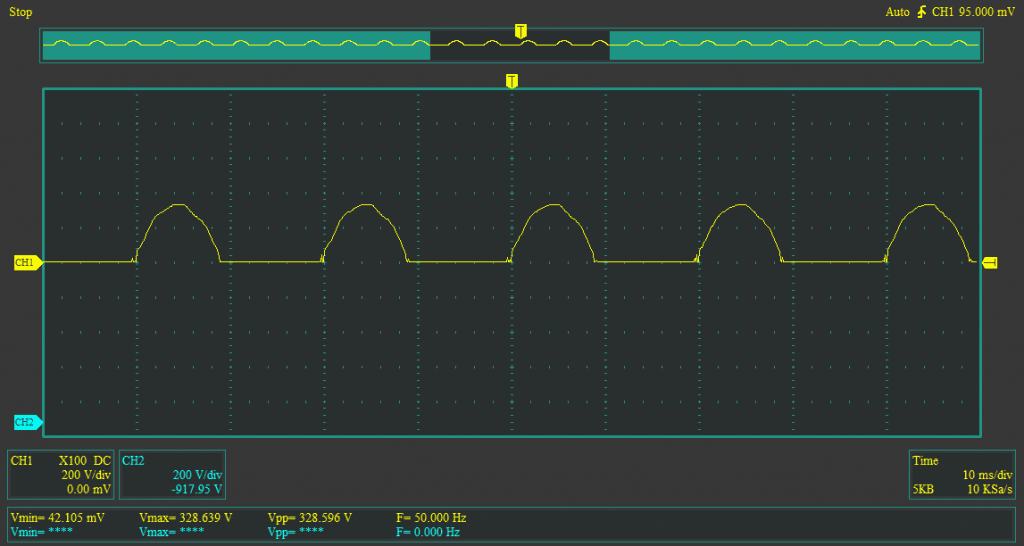The second image is for alpha = 45° (2500 µs):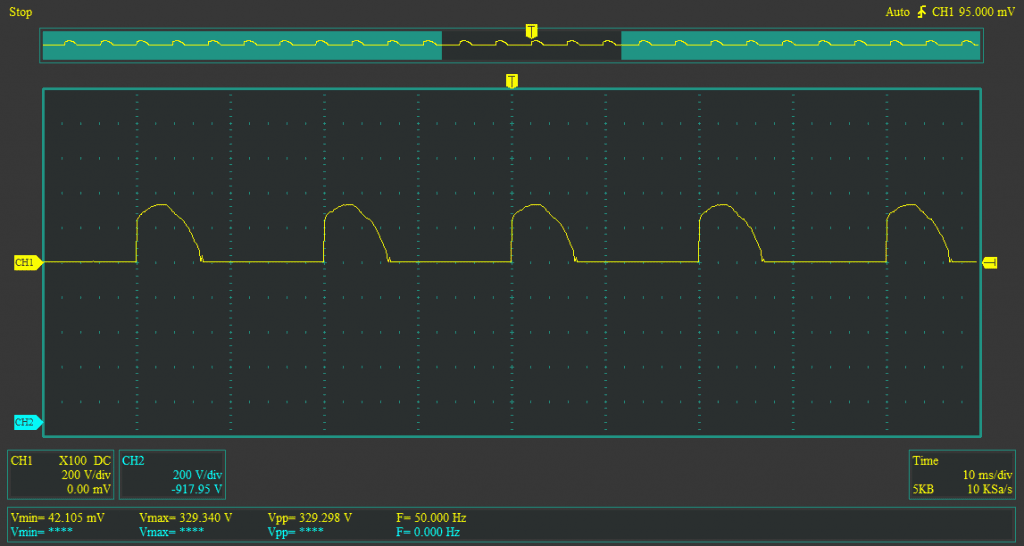This one is for alpha = 90° (5000µs):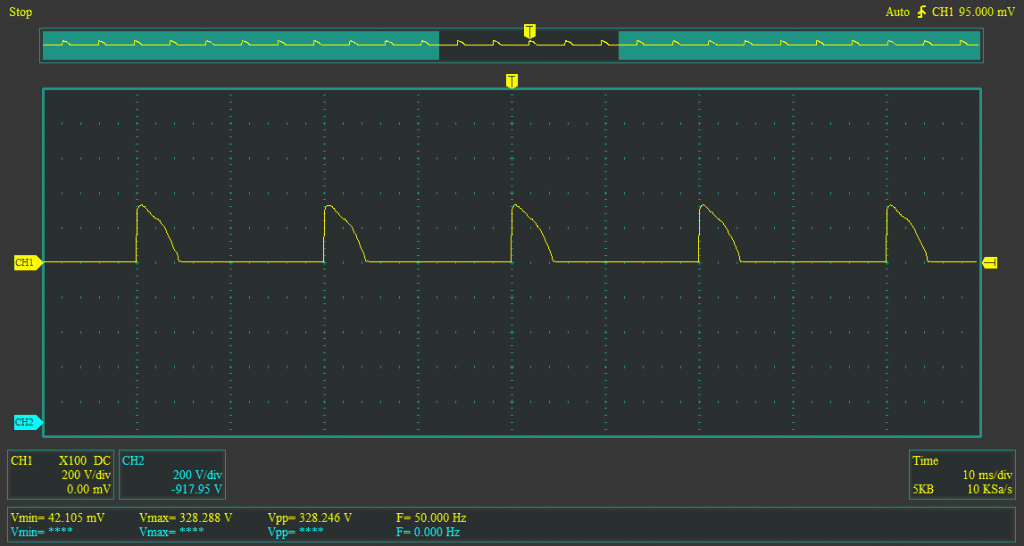And the last result is for alpha = 135° (7500µs):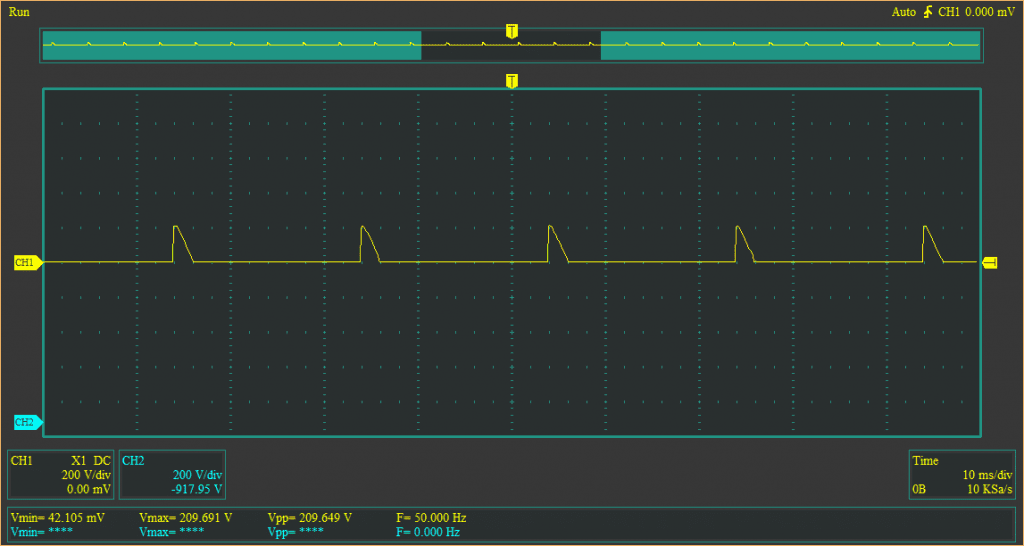Reference:
Power Electronics Handbook – MUHAMMAD H. RASHID

This site uses Akismet to reduce spam. Learn how your comment data is processed.